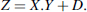## 12.1 NATURAL NUMBERS

### 12.1.1 Basic Multiplier

According to the Hörner expansion presented in Chapter 5, formula (5.6),is easily mapped into a combinational circuit to materialize Algorithm 5.2 (shift and add 2). A basic space iteration of the shift and add multiplier in base B is shown in Figure 12.1. The function Z implemented by this n-digit × m-digit multiplier iswhere D = P(0) is an m-digit number.

Whenever B > 2, the size of the result Z is m + n; moreover, (m + 1)-digit adders are needed, because xi.Y may exceed Bm − 1. Otherwise, in the binary case, the size of the result is limited to m + n − 1, and m-digit adders meet the requirement. After each addition step, a digit result appears as the rightmost digit of the shifted sum. According to the case at hand, inverting the role of multiplicand and multiplicator may appear useful. The effects of this permutation are that the products yi.X are n-digit products (instead of m for xi.Y), while the n (m + 1)-digit adders are switched for m (n + 1)-digit ones. Obviously the size of the result doesn't change.

The hardware cost of this circuit is high because of the n (resp. m) adders involved. The time is roughly equal to n (m + 1)-digit (resp. m (n + 1)-digit) adders. As will be observed later, if ripple-carry adders are used, this ...

Get Synthesis of Arithmetic Circuits: FPGA, ASIC and Embedded Systems now with the O’Reilly learning platform.

O’Reilly members experience books, live events, courses curated by job role, and more from O’Reilly and nearly 200 top publishers.